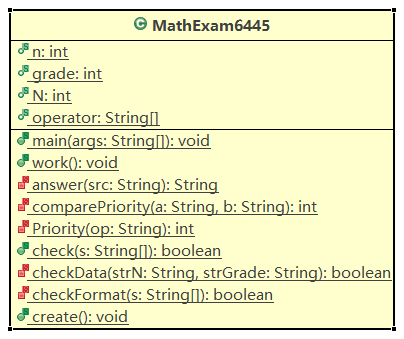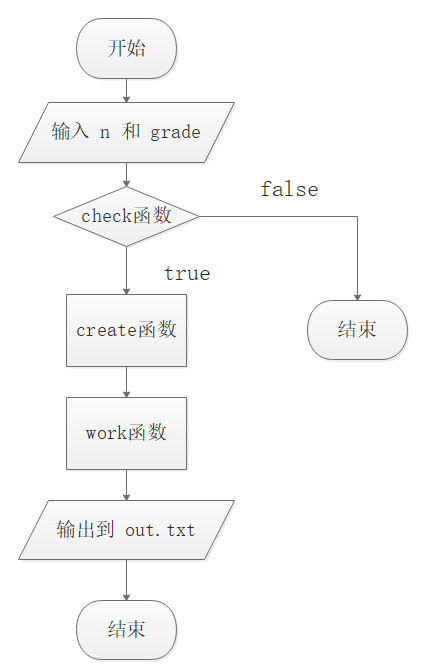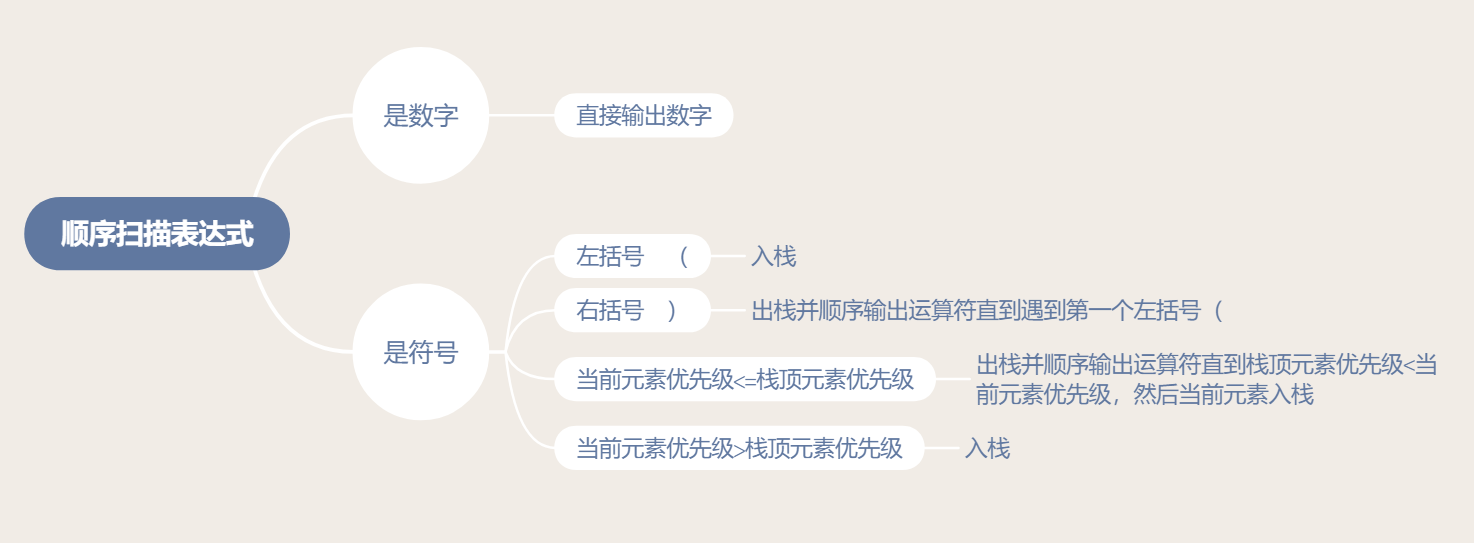# 程序开发——结对编程

#### 结对组合

学号1：211606367 姓名：林恩       学号2：211606445 姓名：肖志豪


## 一、预估与实际

PSP2.1 Personal Software Process Stages 预估耗时（分钟） 实际耗时（分钟）
Planning 计划 10 10
• Estimate • 估计这个任务需要多少时间 10 10
Development 开发 420 750
• Analysis • 需求分析 (包括学习新技术) 30 20
• Design Spec • 生成设计文档 60 20
• Design Review • 设计复审 10 10
• Coding Standard • 代码规范 (为目前的开发制定合适的规范) 10 10
• Design • 具体设计 60 60
• Coding • 具体编码 200 250
• Code Review • 代码复审 20 30
• Test • 测试（自我测试，修改代码，提交修改） 30 350
Reporting 报告 60 50
• Test Repor • 测试报告 40 30
• Size Measurement • 计算工作量 10 10
• Postmortem & Process Improvement Plan • 事后总结, 并提出过程改进计划 10 10

## 二、需求分析

（1）小学一年级数学有如下的几个特点：

• 特点1：掌握100以内加减法

• 特点2：未学习小数和负数

经过分析，我认为，这个程序应当：

• 加法和减法使用的数字小于100（加法计算结果也不能超出）

• 小学还未学习负数（减法计算结果也不能出现负数）

• 小学一年级尚未学习乘除，所以四则运算暂时不做乘除运算。

（2）小学二年级数学新增如下的几个特点：

• 特点1：掌握乘法口诀表

• 特点2：10以内的除法

经过分析，我认为，这个程序更新后应当：

• 加法和减法使用的数字小于100（加法计算结果也不能超出）

• 小学还未学习负数（减法计算结果也不能出现负数）

• 乘法和除法使用的数字小于10（乘法计算结果可以超出）

（3）小学三年级数学新增如下的几个特点：

• 特点1：加减乘除四则混合运算

• 特点2：乘除运算拓展为100以内的乘除

• 特点3：四则运算中除法不能有余数

• 特点4：学习了小括号的使用

• 一二年级的功能保留

• 四则混合运算中运算符在 2～4 个

• 四则混合运算中可以加括号

• 除法运算除数不能为0，不能有余数

• 括号须有意义，并不能是无意义括号

• 运算结果不超过10000

## 三、设计

### 1. 设计思路

这个程序就一个类，九个函数，其中4个为公共的函数，5个为私有函数。main函数接收输入的信息，之后调用check函数检查输入的信息是否有误（其中分别去调用checkFormat函数和checkData函数分别检查输入的信息在格式和数据上是否有误），有误给出提示，无误则继续调用create函数创建out.txt输出文件。最后调用work函数出题和给出答案（调用answer函数）。流程图：### 2. 实现方案

• 准备工作：先在Github上创建仓库，克隆到本地，在eclipse上编写代码。

• 技术关键点：梳理一下设计思路，可能遇到哪些技术关键点

• 命令行参数输入

• 参数正则匹配

• 重定向输出到文件

• 生成四则混合运算式

• 求解四则混合运算式

• 算法：调度场算法与逆波兰表达式。## 四、编码

### 2. 关键代码

/**
* 出题函数
* 根据输入的年级和题数生成对应题目，并打印题目和最后答案
*/
public static void work() {
Random rand = new Random();
// src:题目字符串。 result:答案字符串。lineBreak:换行字符串。
String src = "";
String result = "";
String lineBreak = "\r\n";
// 标记为为外层循坏，以便continue退出。
outerLoop: for (int i = 1; i <= n; i++) {
num1 = rand.nextInt(101);
num2 = rand.nextInt(101);
// 一年级只有加减，所以运算符随机生成数为0和1
op = rand.nextInt(2);
// 符合条件则计算答案
if (op == 0 && num1 + num2 <= 100) {
} else if (op == 1 && num1 - num2 > 0) {
} else {
// 不符合条件则退出本次循环，并i--重新生成这一题
i--;
continue;
}
// 将题目添加到题目字符串，答案添加到答案字符串中
src += "(" + i + ") " + num1 + operator[op] + num2 + lineBreak;
result += "(" + i + ") " + num1 + operator[op] + num2 + " = " + answer + lineBreak;
} else if (grade == 2) {
// 二年级涉及乘除，所以运算符随机生成数为0到3
op = rand.nextInt(4);
if (op < 2) {
// 加减为百以内的加减
num1 = rand.nextInt(101);
num2 = rand.nextInt(101);
} else {
// 乘除为表内乘除，所以取值范围是0到10
num1 = rand.nextInt(11);
num2 = rand.nextInt(11);
}
// 符合条件则计算答案
if (op == 0 && num1 + num2 <= 100) {
} else if (op == 1 && num1 - num2 >= 0) {
} else if (op == 2 && num1 * num2 <= 100) {
} else if (op == 3 && num2 != 0) {
} else {
// 不符合条件则退出本次循环，并i--重新生成这一题
i--;
continue;
}
// 将题目添加到题目字符串中
src += "(" + i + ") " + num1 + operator[op] + num2 + lineBreak;
// 将答案添加到答案字符串中，如果是除法要考虑是否有余数
if (op != 3)
result += "(" + i + ") " + num1 + operator[op] + num2 + " = " + answer + lineBreak;
else {
if (num1 % num2 != 0)
result += "(" + i + ") " + num1 + operator[op] + num2 + " = " + answer + "..." + (num1 % num2)
+ lineBreak;
else
result += "(" + i + ") " + num1 + operator[op] + num2 + " = " + answer + lineBreak;
}
} else {
// 三年级，新增功能
num1 = rand.nextInt(101);
// isBracket随机生成数0和1，用来存储是否有括号，0没有括号，1有括号。
int isBracket = rand.nextInt(2);
if (isBracket == 0) {
// opNum随机生成数2到4，用来存储运算符个数
int opNum = rand.nextInt(3) + 2;
// 定义一个字符串，用来存储这一题的题目生成，初始化为第一个随机生成数
String str = num1 + "";
// 标记为为内层循坏，以便continue退出。
innerLoop: for (int j = 1; j <= opNum; j++) {
op = rand.nextInt(4);
num2 = rand.nextInt(101);
// 不符合题目生成规则则退出本次循环，并j--重新生成运算符和第二个随机生成数
if (op == 0) {
if (num1 + num2 >= 1000) {
j--;
continue innerLoop;
}
} else if (op == 1) {
if (num1 - num2 < 0) {
j--;
continue innerLoop;
}
} else if (op == 2) {
if (answer * num2 > 1000) {
j--;
continue innerLoop;
}
} else if (op == 3) {
if (num2 == 0 || answer % num2 != 0) {
j--;
continue innerLoop;
}
}
// 符合题目生成条件，先计算这步结果，如果这步结果是负数或者超出小学生三年级范围，退到外层循环，并i--重新生成这题
String s = str + operator[op] + num2;
if (num1 < 0 || num1 > 10000) {
i--;
continue outerLoop;
}
// 结果也符合，将这步添加到题目
str += operator[op] + num2;
}
// 最后将生成的题目添加到题目字符串和答案字符串中
src += "(" + i + ") " + str + lineBreak;
result += "(" + i + ") " + str + " = " + answer(str.substring(str.indexOf(')') + 1)) + lineBreak;
} else {
// 最多四个运算符，有括号的情況有：
// （a op b）op c 和 a op（b op c）
// 所以从括号的位置可以分为 在前和在后，随机生成括号位置site，0在前，1在后
int site = rand.nextInt(2);
if (site == 0) {
// 定义一个字符串，用来存储这一题的题目生成，初始化为 "("+第一个随机生成数
String str = "( " + num1;
// 由于括号在前，括号内的运算符只需加减即可，乘除加括号没有意义（都需从左到右计算）
op = rand.nextInt(2);
num2 = rand.nextInt(101);
// 同理，不符合题目生成规则则退出循环，并i--重新生成题目
if (op == 0) {
if (num1 + num2 >= 1000) {
i--;
continue outerLoop;
}
} else {
if (num1 - num2 < 0) {
i--;
continue outerLoop;
}
}
// 符合就添加到str中
str += operator[op] + num2 + " )";
// 括号外边的运算符为乘除，否则括号加了没有意义
op = rand.nextInt(2) + 2;
// 同理，判断是否符合题目生成规则
if (op == 2) {
if (num1 * num2 > 1000) {
i--;
continue outerLoop;
}
} else {
if (num2 == 0 || num1 % num2 != 0) {
i--;
continue outerLoop;
}
}
str += operator[op] + num2;
if (num1 < 0 || num1 > 10000) {
i--;
continue outerLoop;
}
// 将题目和答案分别添加到题目字符串和答案字符串中
src += "(" + i + ") " + str + lineBreak;
result += "(" + i + ") " + str + " = " + answer(str) + lineBreak;
} else {
// 括号在后，逻辑和括号在前类似
op = rand.nextInt(2);
num2 = rand.nextInt(101);
if (op == 0) {
if (num1 + num2 >= 1000) {
i--;
continue outerLoop;
}
} else {
if (num1 - num2 < 0) {
i--;
continue outerLoop;
}
}
String str = "( " + num1 + operator[op] + num2 + " )";
op = rand.nextInt(2) + 2;
num1 = rand.nextInt(101);
if (op == 2) {
if (num1 * num2 > 1000) {
i--;
continue outerLoop;
}
} else {
if (num2 == 0 || num1 % num2 != 0) {
i--;
continue outerLoop;
}
}
str = num1 + operator[op] + str;
if (num1 < 0 || num1 > 10000) {
i--;
continue outerLoop;
}
src += "(" + i + ") " + str + lineBreak;
result += "(" + i + ") " + str + " = " + answer(str) + lineBreak;
}
}
}
}
System.out.println(src);
System.out.print(result);
}

/**
* 解题函数
* 根据给出的题目通过调度场算法和逆波兰表达式的思路得出计算结果
* @param src 参数是带求解的题目字符串
* @return 返回值是一个字符串：最后结果
*/
private static String answer(String src) {
// 定义两个栈，用来存储数字和运算符，即输出栈和符号栈
Stack<String> print = new Stack<String>();
Stack<String> operator = new Stack<String>();
// 去掉题目中的空格
String str = src.replace(" ", "");
// 定义一个number字符串，用来存储数字，不会将数字拆分，例如23不会拆成2和3
String number = "";
// 遍历字符串str，是数字添加到输出栈，是符号就和符号栈栈顶元素比较优先级
for (int i = 0; i < str.length(); i++) {
char c = str.charAt(i);
if (c >= '0' && c <= '9') {
number += c + "";
// 如果到达最后，将number入栈（输出栈），同时将number清空
if (i + 1 >= str.length()) {
print.push(number);
number = "";
}
} else {
// 如果number里有数字，将number入栈（输出栈），同时将number清空
if (!number.isEmpty()) {
print.push(number);
number = "";
}
// 如果当前符号（1）是左括号或者（2）符号栈为空或者（3）当前符号优先级>栈顶符号优先级，直接入栈
if (c == '(' || operator.isEmpty() || comparePriority(c + "", operator.peek()) == 1) {
operator.push(c + "");
} else if (c == ')') {
// 如果当前符号为右括号，需要出栈直到遇到第一个左括号为止。
String stackTop = operator.pop();
while (!stackTop.equals("(")) {
// 出栈的时候，同时进行逆波兰表达式的计算，取出输出栈的前两个数
int number1 = Integer.parseInt(print.pop());
int number2 = Integer.parseInt(print.pop());
int number3;
// 按出栈的符号计算得出结果number3
if (stackTop.equals("+")) {
number3 = number2 + number1;
} else if (stackTop.equals("-")) {
number3 = number2 - number1;
} else if (stackTop.equals("×")) {
number3 = number2 * number1;
} else {
number3 = number2 / number1;
}
// 并将结果入栈（输出栈）
print.push(number3 + "");
stackTop = operator.pop();
}
} else if (comparePriority(c + "", operator.peek()) != 1) {
// 如果当前符号优先级<=栈顶符号优先级，需要出栈直到当前符号优先级>栈顶符号优先级为止
while (!operator.empty() && comparePriority(c + "", operator.peek()) < 1) {
// 同理，出栈的时候，同时进行逆波兰表达式的计算，取出输出栈的前两个数
int number1 = Integer.parseInt(print.pop());
int number2 = Integer.parseInt(print.pop());
int number3;
// 按出栈的符号计算得出结果number3
String stackTop = operator.peek();
if (stackTop.equals("+")) {
number3 = number2 + number1;
} else if (stackTop.equals("-")) {
number3 = number2 - number1;
} else if (stackTop.equals("×")) {
number3 = number2 * number1;
} else {
number3 = number2 / number1;
}
// 并将结果入栈（输出栈）
print.push(number3 + "");
stackTop = operator.pop();
}
// 最后将当前符号入栈（符号栈）
operator.push(c + "");
}
}
}

// 最后需要将符号栈中的符号依次出栈，同时进行逆波兰表达式计算
while (!operator.empty()) {
String stackTop = operator.pop();
int number1 = Integer.parseInt(print.pop());
int number2 = Integer.parseInt(print.pop());
int number3;
if (stackTop.equals("+")) {
number3 = number2 + number1;
} else if (stackTop.equals("-")) {
number3 = number2 - number1;
} else if (stackTop.equals("×")) {
number3 = number2 * number1;
} else {
number3 = number2 / number1;
}
print.push(number3 + "");
}
return print.peek();
}

/**
* 比较运算符优先级方法
* @param a 此参数为其中一个运算符
* @param b 此参数为另一个运算符
* @return 	如果第一个运算符的优先级大于第二个运算符的优先级，返回 1。
* 			如果第一个运算符的优先级等于第二个运算符的优先级，返回 0。
*         	如果第一个运算符的优先级小于第二个运算符的优先级，返回 -1。
*/
private static int comparePriority(String a, String b) {
if (a.equals(b)) {
return 0;
} else if (Priority(a) > Priority(b)) {
return 1;
} else if (Priority(a) < Priority(b)) {
return -1;
} else {
return 0;
}
}

/**
* 查询运算符优先级方法
* @param op 参数为待查询的运算符
* @return 返回运算符的优先级
*/
private static int Priority(String op) {
if (op.equals("×") || op.equals("÷")) {
return 2;
} else if (op.equals("+") || op.equals("-")) {
return 1;
} else {
return 0;
}
}

/**
* 检查函数 检查输入的参数是否符合标准
* @param s 参数是 输入的字符串
* @return 如果输入的参数符合标准，返回true；否则返回false。
*/
public static boolean check(String[] s) {
if (checkFormat(s)) {
if (s.equals("-n"))
return checkData(s, s);
else
return checkData(s, s);
} else {
System.out.println("亲，输入格式错误哦");
return false;
}
}

/**
* 检查数据函数 由check检查函数调用，在检查中起到检查数据的作用。
* @param strN 此参数待检查的题数
* @return 如果题数和年级都符合标准，返回true；否则返回false。
*/
private static boolean checkData(String strN, String strGrade) {
if (!strN.matches("\\d*")) {
if (strN.matches("-\\d*")) {
System.out.println("输入一个正确的数字哦，不能为负");
return false;
} else {
System.out.println("输入的题数必须是数字!");
return false;
}
} else {
n = Integer.parseInt(strN);
if (n > 100) {
System.out.println("输入的题数过大，体谅下小学生哦");
return false;
} else if (n == 0) {
System.out.println("输入的题数为零，那我还要不要出题呀");
return false;
}
}

System.out.println("对不起，亲，目前只有1到3年级哦");
return false;
} else {
}

return true;
}

/**
* 检查格式函数 由check检查函数调用，在检查中起到检查格式的作用。
* @param s 参数为待检查的字符串数组
* @return 如果输入的字符串数组的格式符合标准，返回true；否则返回false。
*/
private static boolean checkFormat(String[] s) {
return true;
} else {
return false;
}
}


### 3. 代码规范

• 第一条：代码中的命名均不能以下划线或美元符号开始，也不能一下划线或美元符号结束。

• 第二条：代码中的命名严禁使用拼音与英文混合的方式，更不允许直接使用中文的方式。

• 第三条：注释的双斜线与注释内容之间有且仅有一个空格。

• 第四条：方法名、参数名、成员变量、局部变量都统一使用 lowerCamelCase 风格，必须遵循驼峰形式。

• 第五条：杜绝完全不规范的缩写，避免忘文不知义。

• 第六条：不允许任何魔法值（即未经预先定义的常量）直接出现在代码中。

• 第七条：大括号的使用约定。如果是大括号内为空，则简介地写成{}即可，不需要换行；如果是非空代码块则：左大括号前不换行。左大括号后换行。右大括号前换行。右大括号后还有 else 等代码则不换行；表示终止的右大括号后必须换行。

• 第八条：左小括号和字符之间不出现空格；同样的，有小括号和字符之间也不出现空格。详见第5条下面正例提示。

• 第九条：循环体内，字符串的连接方法，使用StringBuilder的append方法进行扩展。
并人工检查代码是否符合规范

## 五、测试

• | :-: | -:
java MathExam6445 - n 100 - gr a de 1 | 亲，输入格式错误哦 | 同预期结果
java MathExam6445 -n -grade 100 1 | 亲，输入格式错误哦 | 同预期结果
java MathExam6445 -grade -n 1 100 | 亲，输入格式错误哦 | 同预期结果
java MathExam6445 -n 100 1 -grade | 亲，输入格式错误哦 | 同预期结果
java MathExam6445 -grade 1 100 -n | 亲，输入格式错误哦 | 同预期结果
java MathExam6445 -n 100000 -grade 1 | 输入的题数过大，体谅下小学生哦 | 同预期结果
java MathExam6445 -n -100 -grade 1 |输入一个正确的数字哦，不能为负 | 同预期结果
java MathExam6445 -n 0 -grade 1 | 输入的题数为零，那我还要不要出题呀 | 同预期结果
java MathExam6445 -n xzh -grade 1| 输入的题数必须是数字! | 同预期结果
java MathExam6445 -n 00000000 -grade 1 | 输入的题数为零，那我还要不要出题呀 | 同预期结果
java MathExam6445 -n 00000001 -grade 1 | 通过检查 | 同预期结果
java MathExam6445 -grade 1 -n 100000 | 输入的题数过大，体谅下小学生哦 | 同预期结果
java MathExam6445 -grade 1 -n -100 |输入一个正确的数字哦，不能为负 | 同预期结果
java MathExam6445 -grade 1 -n 0 | 输入的题数为零，那我还要不要出题呀 | 同预期结果
java MathExam6445 -grade 1 -n xzh| 输入的题数必须是数字! | 同预期结果
java MathExam6445 -grade 1 -n 00000000 | 输入的题数为零，那我还要不要出题呀 | 同预期结果
java MathExam6445 -grade 1 -n 00000001 | 通过检查 | 同预期结果
java MathExam6445 -n 100 -grade -1 | 对不起，亲，目前只有1到3年级哦 | 同预期结果
java MathExam6445 -n 100 -grade 0| 对不起，亲，目前只有1到3年级哦 | 同预期结果
java MathExam6445 -n 100 -grade 1| 通过检查 | 同预期结果
java MathExam6445 -n 100 -grade 2| 通过检查 | 同预期结果
java MathExam6445 -n 100 -grade 3| 通过检查 | 同预期结果
java MathExam6445 -n 100 -grade 4| 对不起，亲，目前只有1到3年级哦 | 同预期结果
java MathExam6445 -n 100 -grade xzh| 对不起，亲，目前只有1到3年级哦 | 同预期结果
java MathExam6445 -grade -1 -n 100| 对不起，亲，目前只有1到3年级哦 | 同预期结果
java MathExam6445 -grade 0 -n 100| 对不起，亲，目前只有1到3年级哦 | 同预期结果
java MathExam6445 -grade 1 -n 100| 通过检查 | 同预期结果
java MathExam6445 -grade 2 -n 100| 通过检查 | 同预期结果
java MathExam6445 -grade 3 -n 100| 通过检查 | 同预期结果
java MathExam6445 -grade 4 -n 100| 对不起，亲，目前只有1到3年级哦 | 同预期结果
java MathExam6445 -grade xzh -n 100| 对不起，亲，目前只有1到3年级哦 | 同预期结果

## 六、总结

posted @ 2018-09-19 01:47  Lomen~  阅读(542)  评论(0编辑  收藏  举报